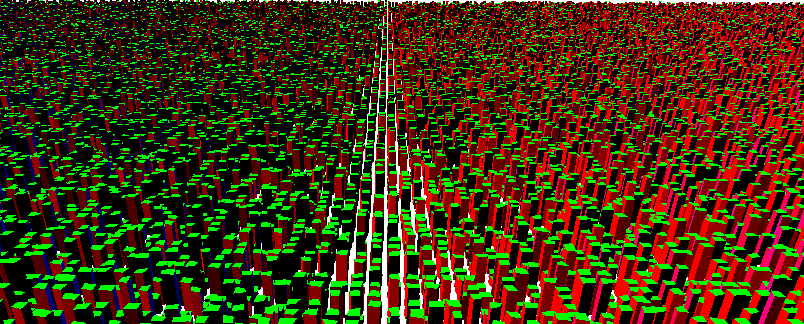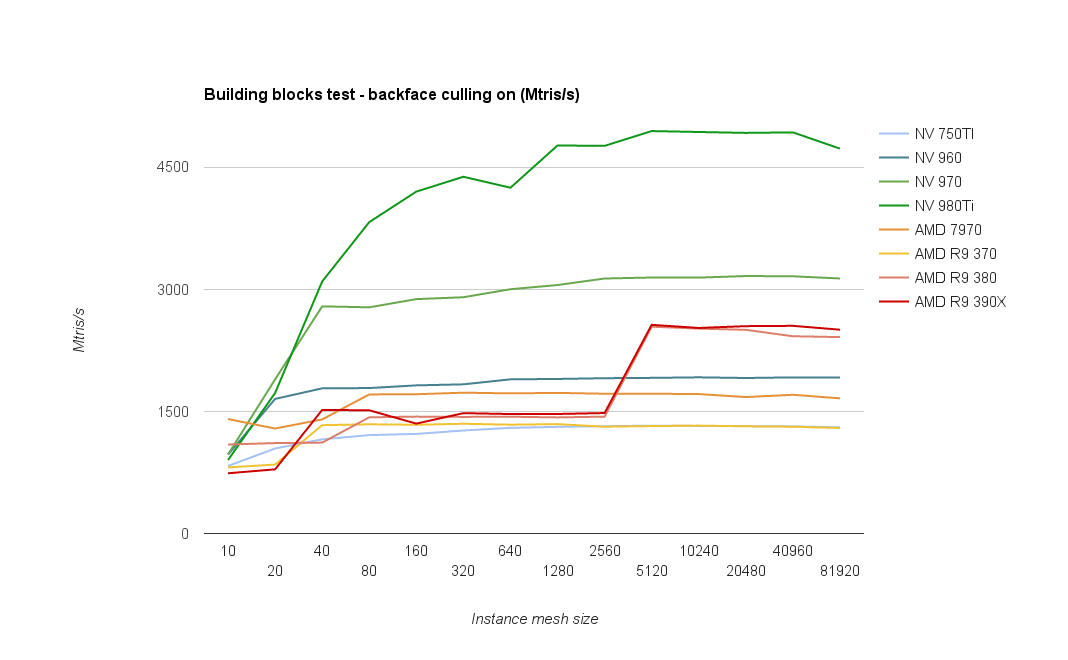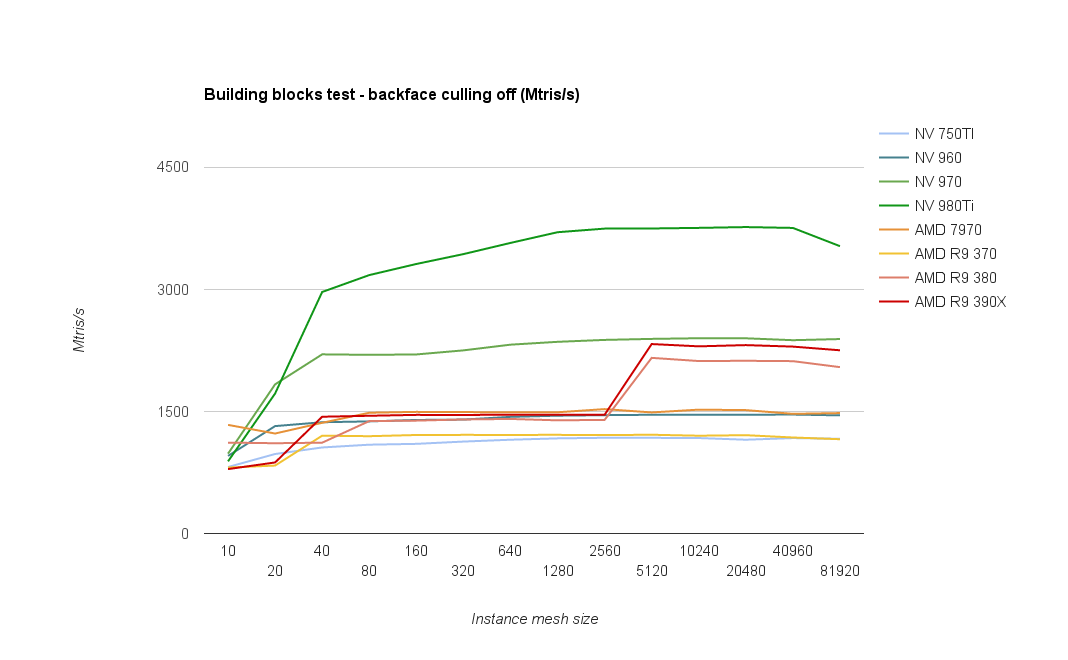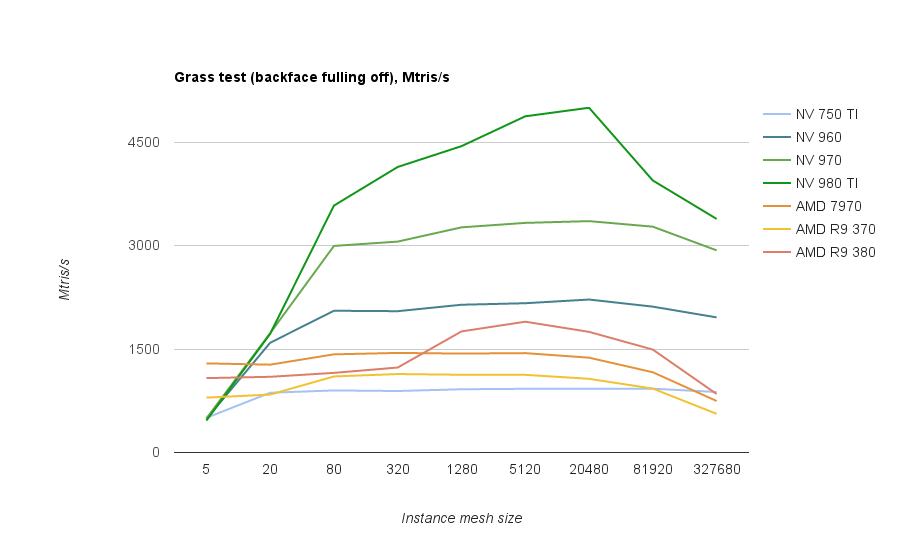## Sunday, January 28, 2018

### Comparison in one of the extremely buggy areas in SRTM 1" (30m), tile s50w075:

Original SRTM 1" (30m) data here:SRTM 1"

SRTM1 here contains lots of errors, from artificial narrow peaks to holes and areas clamped to the sea level, as well as some negative heights.

SRTM3 (90m) dataset handles this area slightly better, with fewer holes, but it contains different linear artifacts and the elevations are sometimes dead wrong anyway, or the voids are filled from extremely coarse data:SRTM 3" (scaled)
This area is full of SRTM voids, places where the radar could not get reliable info due to clouds or other factors, and these holes were filled from various other datasets.

NasaDEM is supposed to be a new reprocessing of raw SRTM data, also using newer sources for void fills. Unfortunately, the preliminary dataset leaves a lot to be desired:NasaDEM (preliminary)
While it's considerably better than SRTM1 in this area, there are still severe artifacts that show up as very ugly sharp transitions in 3D, and it's basically unusable without extensive corrections.

For these corrections it's possible to use another global dataset that is without (significant) artifacts, Viewfinder Panoramas with a global 3" coverage. This one fills SRTM voids using various local maps.Viewfinder 3" (scaled)

### Comparison in previously OK area - river Amazon delta n00w051SRTM 1"
We checked this area only because of a slight mismatch between elevations and the water mask, indicating that perhaps the water mask was acquired at a later date and the terrain changed. To our surprise, NasaDEM filling algorithm introduced a new issue - elevation now goes into negative numbers:NasaDEM (preliminary)

So far it seems that the new dataset won't solve our existing problems and will introduce some new ones, although this is all preliminary and unofficial.

## Tuesday, June 13, 2017

### fp64 approximations for sin/cos for OpenGL

Minimax approximations for missing fp64 functions in GLSL, using remez exchange toolbox. For additional details see Double precision approximations for map projections in OpenGL.

//sin approximation, error < 5e-9
double sina_9(double x)
{
//minimax coefs for sin for 0..pi/2 range
const double a3 = -1.666665709650470145824129400050267289858e-1LF;
const double a5 =  8.333017291562218127986291618761571373087e-3LF;
const double a7 = -1.980661520135080504411629636078917643846e-4LF;
const double a9 =  2.600054767890361277123254766503271638682e-6LF;

const double m_2_pi = 0.636619772367581343076LF;
const double m_pi_2 = 1.57079632679489661923LF;

double y = abs(x * m_2_pi);
double q = floor(y);

double t = (quadrant & 1) != 0 ? 1 - y + q : y - q;
t *= m_pi_2;

double t2 = t * t;
double r = fma(fma(fma(fma(a9, t2, a7), t2, a5), t2, a3), t2*t, t);

r = x < 0 ? -r : r;

return (quadrant & 2) != 0 ? -r : r;
}

//sin approximation, error < 2e-11
double sina_11(double x)
{
//minimax coefs for sin for 0..pi/2 range
const double a3 = -1.666666660646699151540776973346659104119e-1LF;
const double a5 =  8.333330495671426021718370503012583606364e-3LF;
const double a7 = -1.984080403919620610590106573736892971297e-4LF;
const double a9 =  2.752261885409148183683678902130857814965e-6LF;
const double ab = -2.384669400943475552559273983214582409441e-8LF;

const double m_2_pi = 0.636619772367581343076LF;
const double m_pi_2 = 1.57079632679489661923LF;

double y = abs(x * m_2_pi);
double q = floor(y);

double t = (quadrant & 1) != 0 ? 1 - y + q : y - q;
t *= m_pi_2;

double t2 = t * t;
double r = fma(fma(fma(fma(fma(ab, t2, a9), t2, a7), t2, a5), t2, a3),

t2*t, t);

r = x < 0 ? -r : r;

return (quadrant & 2) != 0 ? -r : r;
}

Cos can be just offset by π/2

//cos approximation, error < 5e-9
double cosa_9(double x)
{
//sin(x + PI/2) = cos(x)
return sina_9(x + DBL_LIT(1.57079632679489661923LF));
}

//cos approximation, error < 2e-11
double cosa_11(double x)
{
//sin(x + PI/2) = cos(x)
return sina_11(x + DBL_LIT(1.57079632679489661923LF));
}

## Saturday, February 6, 2016

### OpenGL rendering performance test #2 - blocks

Previous test - Procedural grass - focused on procedurally generated geometry that didn’t use any vertex data and generated meshes procedurally using only gl_VertexID value and a few lookups into shape textures, therefore with only a neglectable amount of non-procedural data fetched per instance.This test uses geometry generated from some per-instance data (i.e each produced vertex uses some unique data from buffers), but still partially procedurally generated. Source stream originally consisted of data extracted from OpenStreetMap maps - building footprints broken into quads, meaning there’s a constant size block of data pertaining to one rendered building block (exactly 128 bytes of data per block). Later the data were replaced with a generated content to achieve higher density for the purpose of the testing, but there’s still the same amount of data per block..

Another significant difference is that backface culling is enabled here. With grass the blades are essentially 2D and visible from both sides, and that means that the number of triangles sent to the GPU equals the number of potentially visible triangles, minus the blades that are off the frustum.

In case of the building block test there’s approximately half of the triangles culled away, being back-facing triangles of the boxes. The test measures number of triangles sent in draw calls, but for direct comparison with the grass test we’ll also use the visible triangle metrics.

Here are the results with backface culling enabled:(Note this graph is missing AMD Fury cards, we’d be grateful if anyone having one of these could run the test (link at the end of the blog) and submit the results to us.)

What immediately catches attention is the large boost happening on newer AMD architectures upon reaching 5k triangles per instanced draw call. A boost in that region was visible also in the grass test, though it was more gradual.
No idea what’s happening there, or rather why the performance is so low on smaller sizes. Hopefully AMD folks will be able to provide some hints.

As it was visible on the grass test as well, AMD 380/390 chips perform rather poorly on the smallest meshes (less than 40 triangles), which also corresponds to the relatively poor performance of geometry shaders.

To be able to compare peak performance with the grass test we need to turn off backface culling, getting the following results:With culling off, the GPU has to render all triangles, twice as much as in previous test. It shows on Nvidia cards, taking roughly a 30% hit.
On AMD the dip is much smaller though, indicating that maybe there’s another bottleneck.

Grass test results for comparison (note slightly different instance mesh sizes). Grass test uses much less per-instance data, but has a more complex vertex shader. Apparently, it suits Nvidia cards better:Conclusion: both vendors show the best performance when the geometry in instanced calls is grouped into 5 - 20k triangles. On AMD GCN 1.1+ chips the 5k threshold seems to be critical, with performance almost doubling.

## Test sources and binaries

All tests can be found at https://github.com/hrabcak/draw_call_perf

There are 3 tests: grass, buildings and cubes. The tests can be launched by running their respective bat files. Each test run lasts only 4-5 seconds, but there are many tested combinations so the total time can be up to 15 minutes.

Once each test completes, you will be asked to submit the test results to us. The test results are stored in a CSV file, and include the GPU type, driver version and performance measurements.
We will be updating the graphs to fill the missing pieces.